# Battery Monitor

Product: Battery Monitor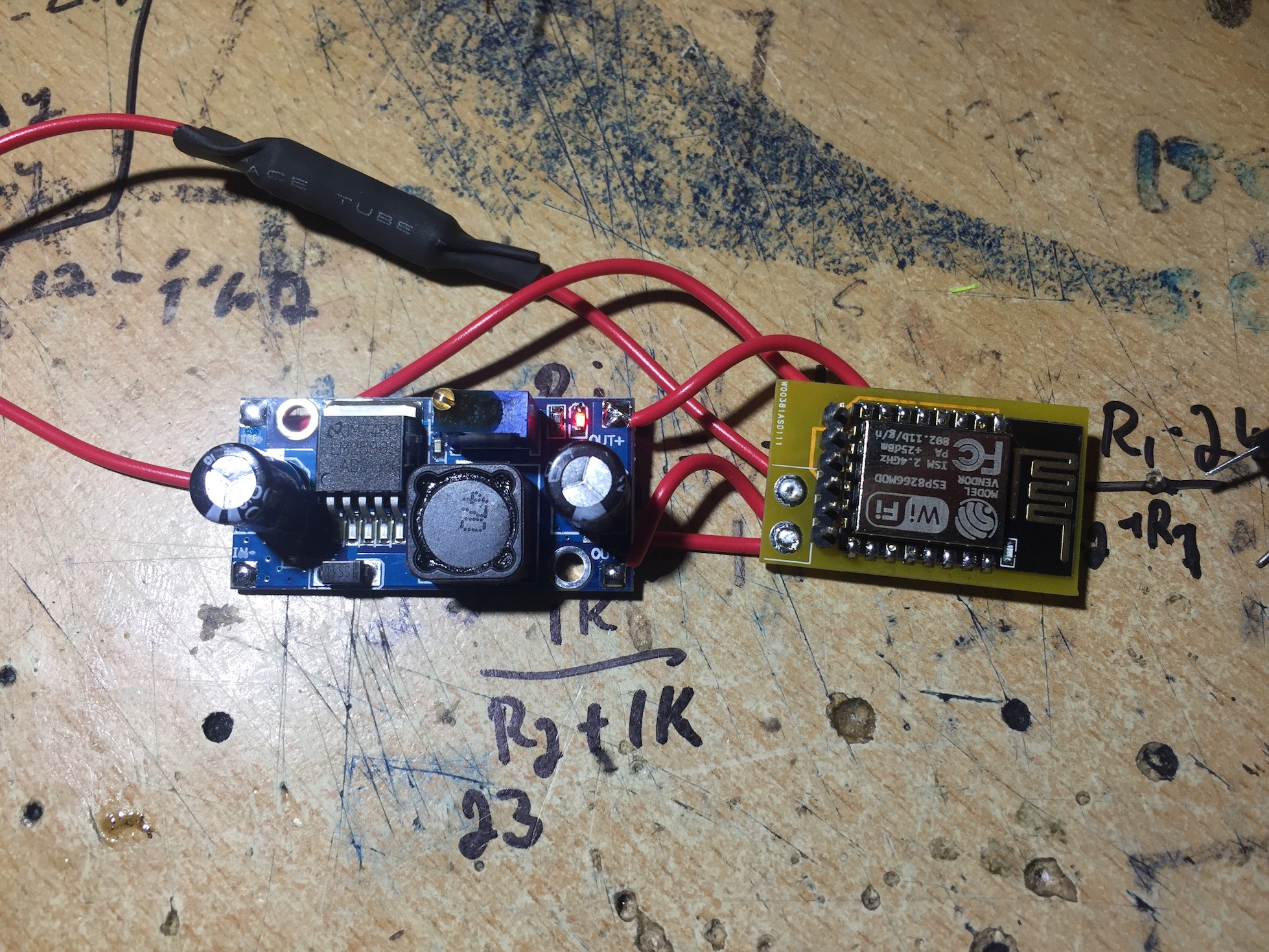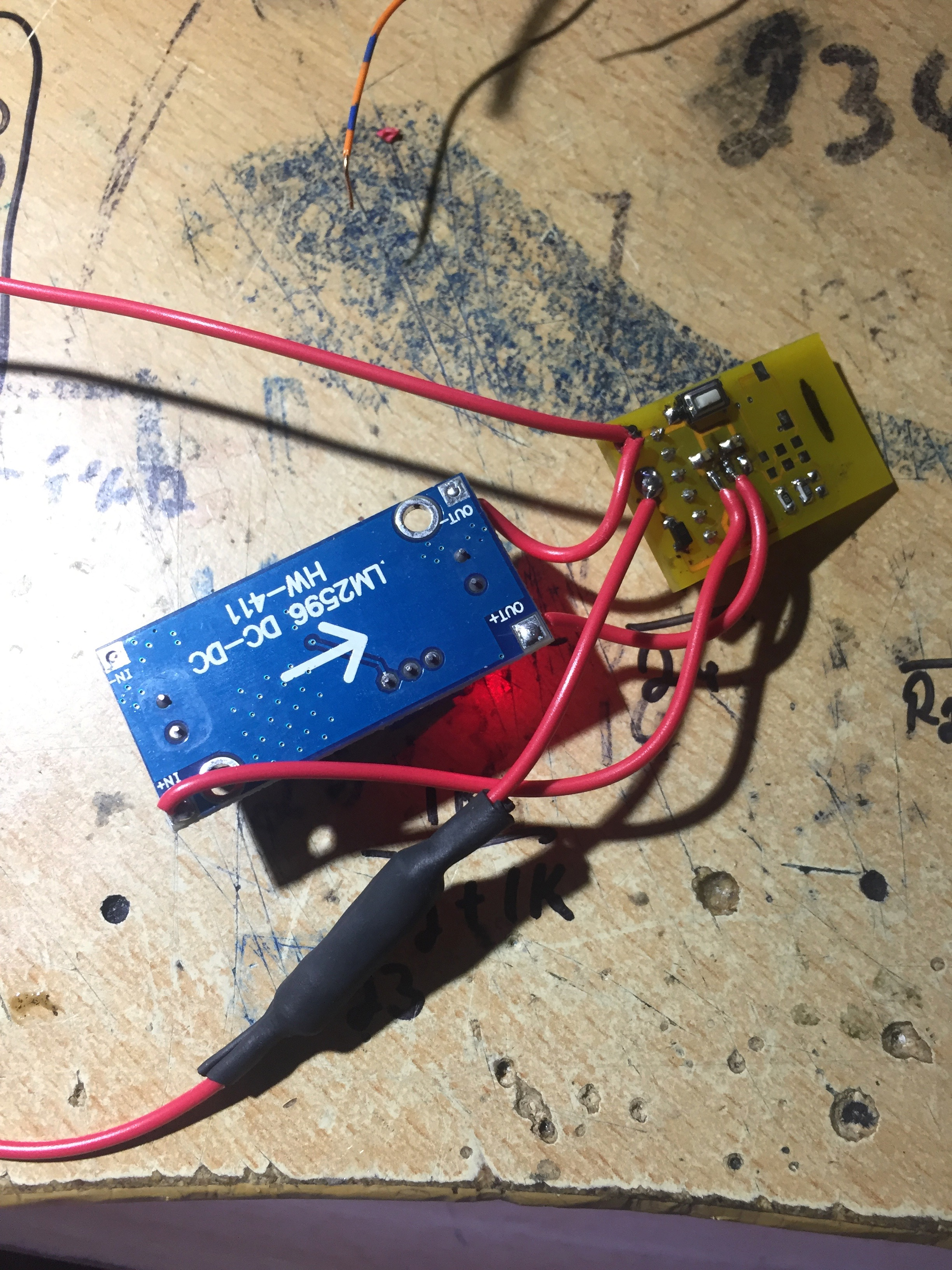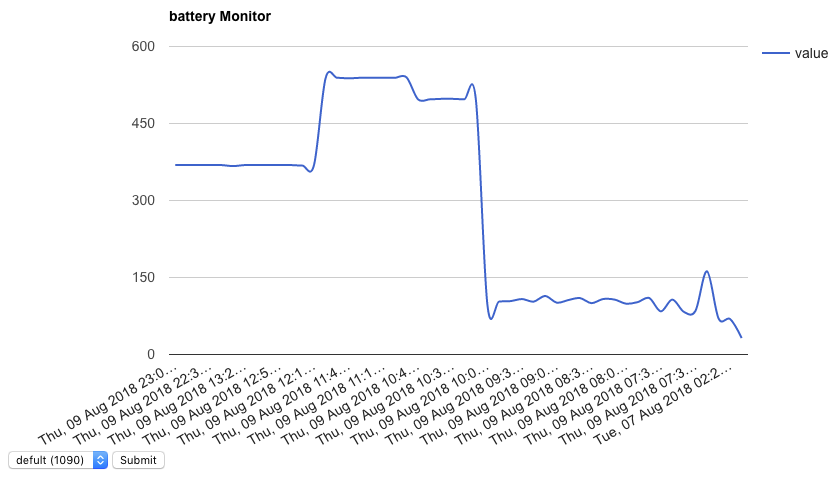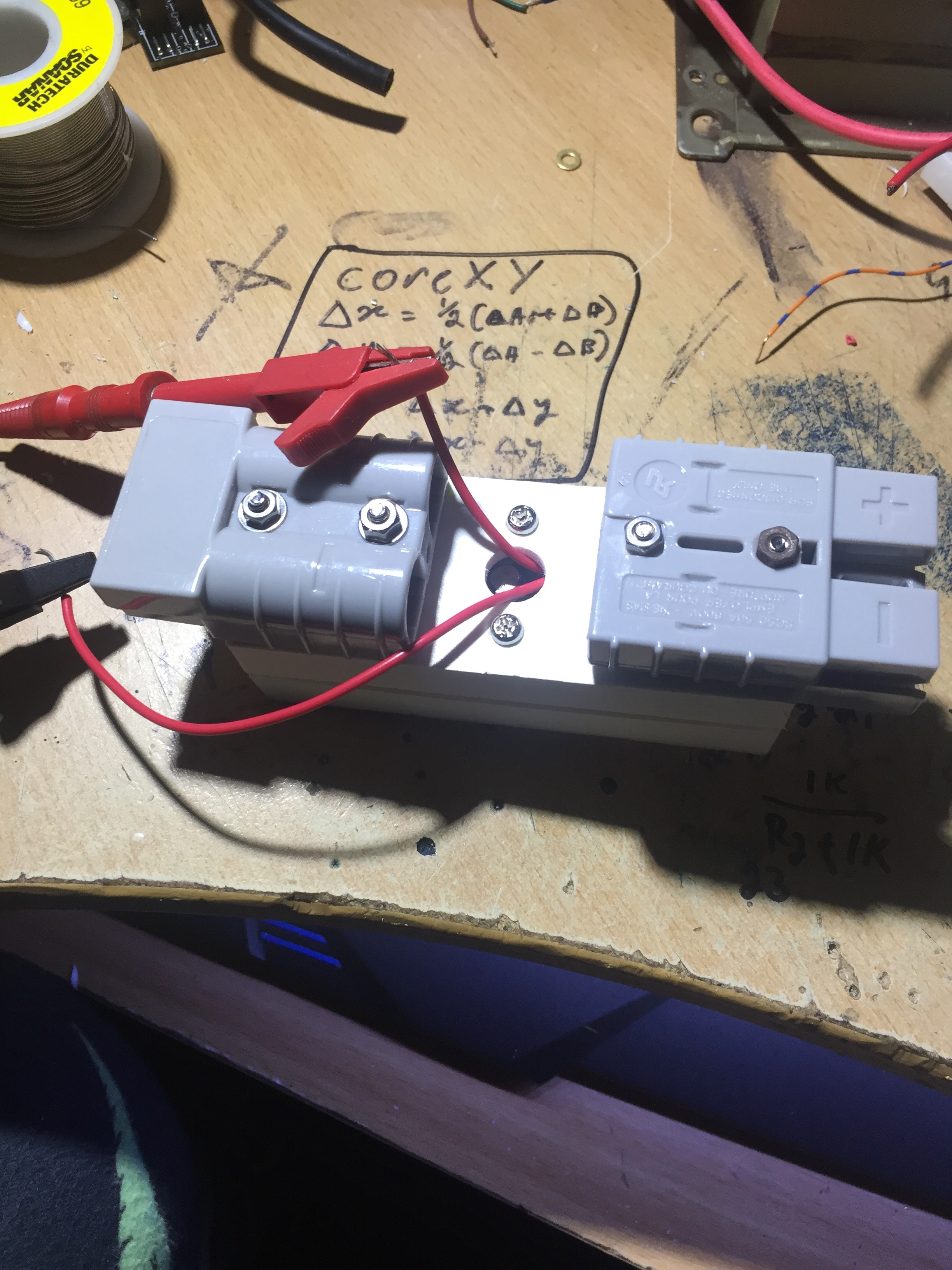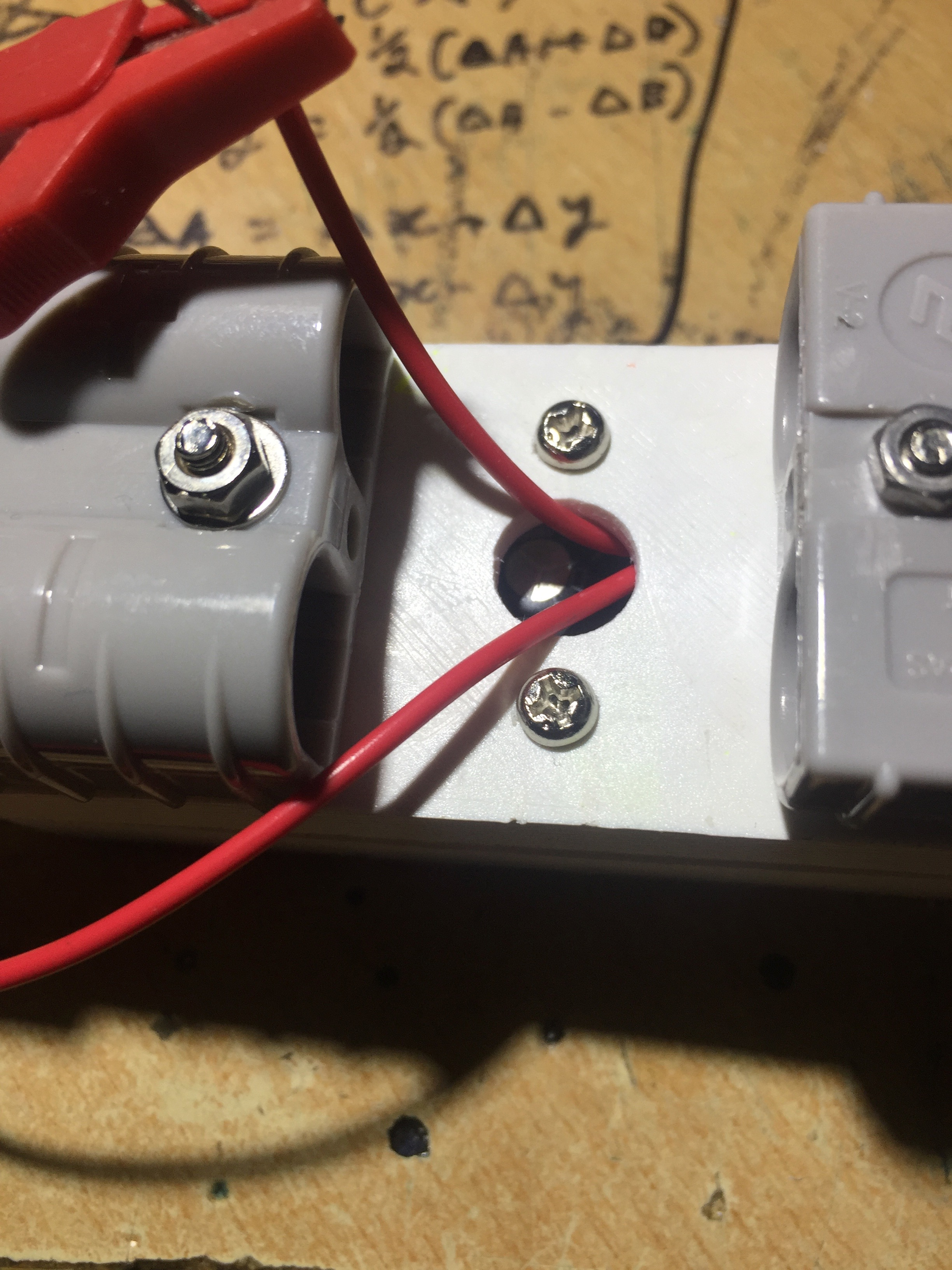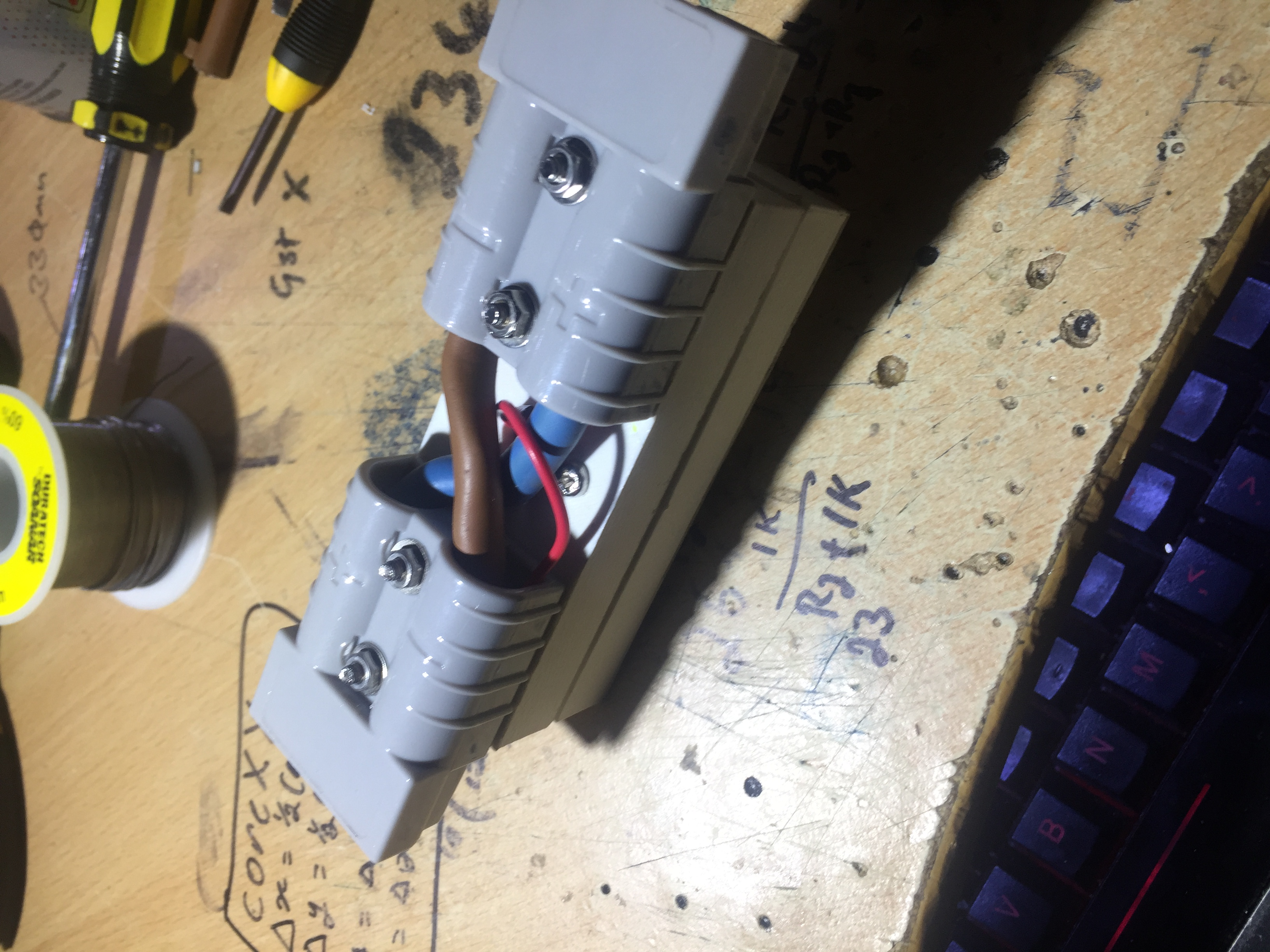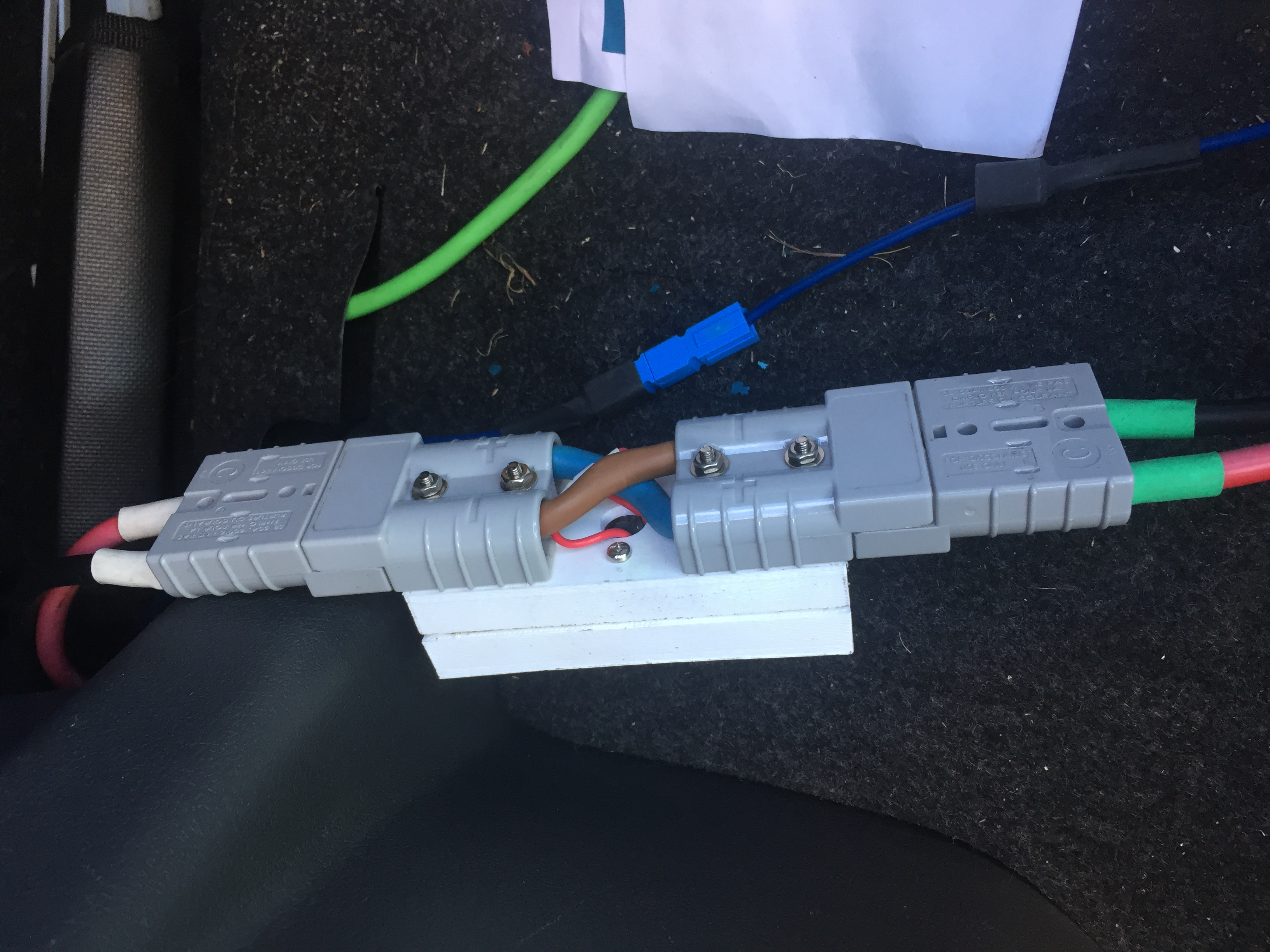This is Wifi battery monitor that can log/store battery voltage and send the data to a database. I made this to try and debug a power problem within my car. I think the alternator is not charging the battery properly or the battery is not accepting the charge. This battery monitor will help keep track of the voltages while the car is off and running.
The battery monitor works by getting the battery voltage and send it to the database. If the monitor is offline, the device will store the voltage against the time of the reading and will attempt to resend it later. The battery monitor can log up to 3 days before starting to overwrite the oldest logged data. The Logged data is a value between 0 and 1024. By using the ohms law, the data can be converted into a voltage value across a resistor. The ADC in the ESP-12E can only read voltages from 0V to 1V. To allow the voltage to be read a voltage divider needed to be used to convert max 24V to 1V. 24V was chosen because battery voltage can spike up and 24V would be a safe voltage to regulate from.

## Code for Project

```#include <ESP8266WiFi.h>          //https://github.com/esp8266/Arduino

//needed for library
#include <DNSServer.h>
#include <ESP8266WebServer.h>
#include <WiFiManager.h>         //https://github.com/tzapu/WiFiManager
#include <ESP8266HTTPClient.h>

#define NumberOfItems 600
#define timeBetweenLog 600 //10 mins or 600 secs
#define avg false //avg on and sleeping off

String date = "";
unsigned long startingTime;
int count = -2;
struct log {unsigned long time;int value;} logItems[NumberOfItems];

unsigned long AvgCount = 0;
unsigned long Avgsum = 0;

String MonitorId="defult";

void setup()
{
Serial.begin(115200);

Serial.println("started");
WiFiManager wifiManager;
WiFiManagerParameter custom_MonitorId("MonitorNameId", "Monitors Name ID", "defult", 40);
wifiManager.autoConnect("Voltage Logger");
MonitorId = String(custom_MonitorId.getValue());

Serial.println("connected...yeey :)");
date = getDate();

startingTime = millis()/1000;
}

void loop()
{
getdata();

if(count>-1)
postData();
//else if(!avg && timeBetweenLog + startingTime - millis() > 10000)
//ESP.deepSleep((timeBetweenLog + startingTime - millis()-10000) * 1000);
}

void getdata()
{
if(avg)
{
AvgCount++;
Avgsum -= Avgsum / AvgCount;
}

if(count == -2 || timeBetweenLog + startingTime < millis()/1000)
{
count++;
if(count >= NumberOfItems)
{
for(int i = 1; i < NumberOfItems; i++)
{
logItems[i-1].time = logItems[i].time;
logItems[i-1].value = logItems[i].value;
}
count--;
}
if(count<0)count = 0;
logItems[count].time = millis()/1000;
if(avg)
{
logItems[count].value = AvgCount;
}
else
{
}

startingTime = millis()/1000;
}
}

void postData()
{
String theDate="";
String time = String(logItems[count].time);
String value = String(logItems[count].value);

HTTPClient http;
http.begin("batterymonitor.php?monitorid=" + MonitorId + "&date=" + date + "&time=" + time + "&value=" + value);
int httpCode = http.GET();
if(httpCode > 0)
{
Serial.printf("[HTTP] GET... code: %d\n", httpCode);
if(httpCode == HTTP_CODE_OK)
{
count--;
}
}
else
{
Serial.printf("[HTTP] GET... failed, error: %s\n", http.errorToString(httpCode).c_str());
}

http.end();
}

String getDate()
{
String theDate="";
HTTPClient http;
http.begin("batterymonitor.php?gettime=1"); //HTTP
int httpCode = http.GET();
if(httpCode > 0)
{
Serial.printf("[HTTP] GET... code: %d\n", httpCode);

if(httpCode == HTTP_CODE_OK)
{
theDate = http.getString();
Serial.println(theDate);
}
}
else
{
Serial.printf("[HTTP] GET... failed, error: %s\n", http.errorToString(httpCode).c_str());
}

http.end();

if(theDate != "") return theDate;
delay(60000);
return getDate();
}```